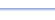Program: TRIAREA

TRIAREA

• Computes the area of a triangle, given three side lengths or two side lengths and the included angle.

Useful for:

• SAT
• ACT
• Geometry
• Precalculus
• Trigonometry
• Calculus

See full list of programs

Example

Q: Find the area of the triangle:

Solution:

Run TRIAREA.  How?

Select choice 1 for
'SAS' because we
are given two side
lengths and the
included angle.

Enter the values
for the side lengths
and angle measure.

The program reports:

Interpretation: The area is about 11.5 square units.